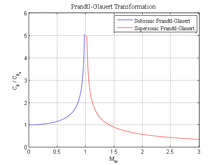# Prandtl–Glauert singularity

﻿
Prandtl–Glauert singularity

The Prandtl–Glauert singularity is the prediction by the Prandtl–Glauert transformation that infinite pressure conditions would be experienced by an aircraft as it approaches the speed of sound. Because it is invalid to apply the transformation at these speeds, the predicted singularity does not emerge. This is related to the early 20th century misconception of the impenetrability of the sound barrier.

## Prandtl-Glauert TransformationPlot of the Prandtl-Glauert transformation as a function of Mach number. Notice the infinite limit at Mach 1.

The Prandtl-Glauert transformation is found by linearizing the potential equations associated with compressible, inviscid flow. It was discovered that the linearized pressures in such a flow were equal to those found from incompressible flow theory multiplied by a correction factor. This correction factor is given below:$c_{p} = \frac {c_{p0}} {\sqrt {|1-{M_{\infty}}^2|}}$

where

This correction factor works well up to low-transonic Mach numbers (M < ~0.7). However, note the limit:$\lim_{M_{\infty} \to 1 }c_p = \infty$

This obviously nonphysical result (of an infinite pressure) is known as the Prandtl-Glauert singularity.

Wikimedia Foundation. 2010.

### Look at other dictionaries:

• Prandtl–Glauert transformation — The Prandtl–Glauert transformation or Prandtl–Glauert rule (also Prandtl–Glauert–Ackeret rule) is an approximation function which allows to compare aerodynamical processes occurring at different Mach numbers. Mathematical expression Even in… …   Wikipedia

• Singularite de Prandtl-Glauert — Singularité de Prandtl Glauert Un McDonnell Douglas F/A 18 Hornet volant à vitesse supersonique. Le nuage est dû à la singularité de Prandtl Glauert …   Wikipédia en Français

• Singularité de prandtl-glauert — Un McDonnell Douglas F/A 18 Hornet volant à vitesse supersonique. Le nuage est dû à la singularité de Prandtl Glauert …   Wikipédia en Français

• Singularité de Prandtl-Glauert — Un McDonnell Douglas F/A 18 Hornet volant à vitesse supersonique. Le nuage est dû à la singularité de Prandtl Glauert …   Wikipédia en Français

• Singularity — may refer to any of a variety of concepts.Mathematics:* Mathematical singularity, a point at which a given mathematical object is not definedIn complex analysis:* Essential singularity, a singularity near which a function exhibits extreme… …   Wikipedia

• Ludwig Prandtl — Infobox Scientist name = Ludwig Prandtl |200px caption = Ludwig Prandtl birth date = birth date|1875|2|4|df=y birth place = Freising, Germany death date = death date and age|1953|8|15|1875|2|4|df=y death place = Göttingen, Germany residence =… …   Wikipedia

• Hermann Glauert — Hermann Glauert, FRS (b. 4 October 1892 in Sheffield, d. 6 August 1934 in Aldershot), was a British aerodynamicist and Principal Scientific Officer of the Royal Aircraft Establishment, Farnborough until Dec 1934. The tragic and incalculable… …   Wikipedia

• Transonic — is an aeronautics term referring to a range of velocities just below and above the speed of sound (about mach 0.8–1.2). It is defined as the range of speeds between the critical mach number, when some parts of the airflow over an aircraft become… …   Wikipedia

• Space Shuttle — STS redirects here. For other uses, see STS (disambiguation). This article is about the NASA Space Transportation System vehicle. For the associated NASA STS program, see Space Shuttle program. For other shuttles and aerospace vehicles, see… …   Wikipedia

• Contrail — Vapor Trail redirects here. For other uses, see Vapor Trail (disambiguation). For other uses, see Contrail (disambiguation). Cirrus aviaticus Contrails from a S7 Airlines Tupolev Tu 154M …   Wikipedia